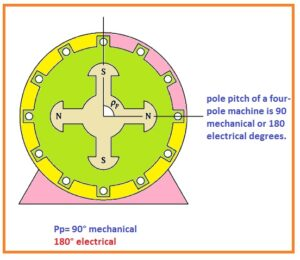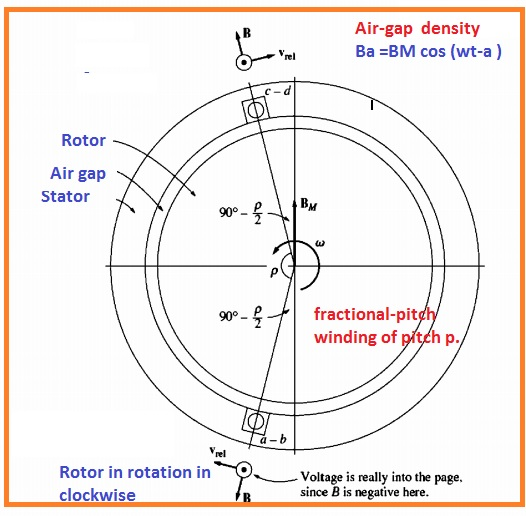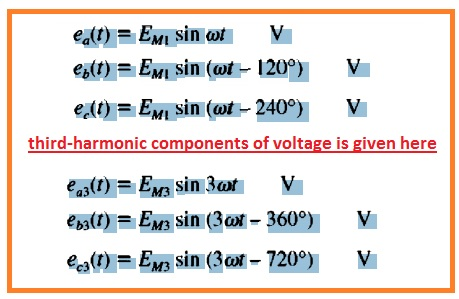There are different types of machines are used in the electrical power system. Like ac generator ac motor is further divided in different types like synchronous generator, motor and induction motor, and induction generator.

In this post, we discuss the winding used in these machines that is pitch and its different factors and outcomes and finds related expression to understand the behavior of coil pitch on the ac machines. So let get started with

### Effect of Coil Pitch On Ac Machines

• In normal ac machines, the value of voltage generated at stator winding is sine waveform due to the flux distributed has sine-like arrangements.
• If the flux density is not like sine-like configured then output voltages are not sine waveform.
• In a practical way, the air gap flux density is not sine-like arrangments but the manufacturer try to make this assembly as like a sine waveform.
• But in reality, there is harmonics elements also exist in the sine distribution of flux.
• due to these harmonics, there is harmonics elements produced in the voltage values existing at stators.
• the generation of harmonics at the voltage and current existing at stator is not good to remove these harmonics different method are used.
• Like this method there one technique used is fractional pitch windings

### What is the Pitch of Coil

• The angular distance among the 2 close pleased poles of any machine is called the pitch of the pole.

Pp=360/p

• Here Pp denoted the pole pitch in mechanical degrees and P denote the number of poles in the machine.
• Irrespective the of poles at machine pole pitch is has value of one eighty degreeFull Pitch Coil

• If the stator windings placed like a similar angle as pole pitch called a full-pitch winding.

fractional-pitch coil

• If the stator windings placed in a shape the angle less than the pole pitch it called fractional pitch winding.
• The pitch for fractional pitch windings is defined as a fraction denoted the part of pole pitch.
• For instance, 5/6 pitch winding space is the distance of two close place poles of five by sixth.
• the pitch of fractional winding in electrical degrees is mentioned here.

P=θm/pp x180

• θm is the angle mechanical angle observed by the winding in degrees and Pp is pole pitch of machine in mechanical degrees
• P=θmP/2 x 180
• Here θm is the mechanical angle in degree and P is the number of poles in motors.

### How Voltage induced in Fractional-Pitch Coil

• To study the effect of fractional pitch at the output voltage we discuss the machine having 2 poles fractional pitch.• For this machine, pole pitch is one-eighty degree and coil pitch is P.
• the voltage induced in this winding through rotating the field can be discussed by finding voltage at every part of the winding.
• the net voltage will be equal to the addition of voltage at all sides.
• Let us suppose that value of flux in air gap among the rotor and stator changes in sine-like arrangements according to mechanical angle and Field is directed in a radical way.
• If α is angle find from the point of extreme rotor flux then the value of flux for any point about the rotor is given here.

B = BM cosα

• As the rotor is moving in the stator with an angular speed of wm so the value of flux density about the stator is mentioned here.

B =BM cos (wt-a )

• The equation for induced voltage in the wire is mentioned here.

eind = (v x B).l

• Here V is velocity
• B is flux
• l is length o the conductor
•  this expression can be used for the static magnetic fields. Let us assume that someone is sitting at the field then it looks like static
• Diagram  indicates the vector field and velocity through view location of static field and wire is in motion### Harmonic Problems in Fractional-Pitch Windings

• the use of practical pitch windings is very good to use in machine sine it described the non sine waveform flux behavior in machine.
• To understand this behavior we see the below figure• In this diagram, there is a synchronous machine used having salient pole rotor
• As the reluctance of field is directed to the mid of rotor then to the sides the flux in existed at point flux value is large.
• The resultant flux generated can see above.
• Note that its shape is not lke sine and has a large number of harmonics
• Since the resultant voltage signal has symmetrical behavior at the mid of rotor flux so there is no harmonics exist in-phase voltage.
• Though all odd harmonics exist in the phase voltage to a certain point.
• in normal the larger the quantity of given harmonic frequency elements the less the magnitude in-phase output.
• When 3 phases are linked in delta and wye configurations certain portion of the harmonic will be diminished through the output.
• The third harmonic elements is given hereThat is a detailed post about the Coil Pitch and Its Effect on AC Machine if you have any further query ask in the comments. Thanks for reading have a nice day